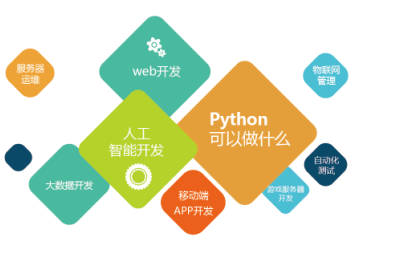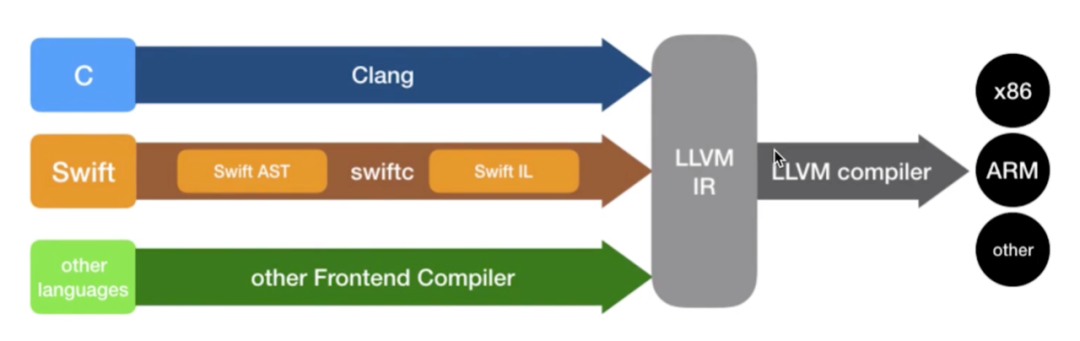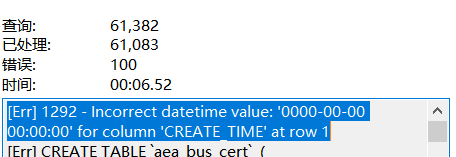Go 函数是“一等公民”的理解

Go 语言以“成为新一代系统级语言”为目标而诞生，但在演化过程中，逐渐演化成了面向并发、契合现代硬件发展趋势的通用编程语言。Go 语言中没有那些典型的面向对象语言的语法，比如类、继承、对象等。Go 语言中的方法（method）本质上亦是函数的一个“变种”。因此，在 Go 语言中，函数是唯一一种基于特定输入、实现特定任务并可反馈任务执行结果的代码块。本质上我们可以说 Go 程序就是一组函数的集合。

• 以“func”关键字开头；
• 支持多返回值；
• 支持具名返回值；
• 支持递归调用；
• 支持同类型的可变参数；
• 支持 defer，实现函数优雅返回

## 1. 什么是“一等公民”

### 1.1 正常创建

``````// \$GOROOT/src/fmt/print.go
func newPrinter() *pp {
p := ppFree.Get().(*pp)
p.panicking = false
p.erroring = false
p.wrapErrs = false
p.fmt.init(&p.buf)
return p
}``````

### 1.2 在函数内创建

``````// \$GOROOT/src/runtime/print.go
func hexdumpWords(p, end uintptr, mark func(uintptr) byte) {
p1 := func(x uintptr) {
var buf [2 * sys.PtrSize]byte
for i := len(buf) - 1; i >= 0; i-- {
if x&0xF < 10 {
buf[i] = byte(x&0xF) + '0'
} else {
buf[i] = byte(x&0xF) - 10 + 'a'
}
x >>= 4
}
gwrite(buf[:])
}
... ...
}``````

### 1.3 作为类型

``````// \$GOROOT/src/net/http/server.go
type HandlerFunc func(ResponseWriter, *Request)

// \$GOROOT/src/sort/genzfunc.go
type visitFunc func(ast.Node) ast.Visitor

// codewalk: https://tip.golang.org/doc/codewalk/functions/
type action func(current score) (result score, turnIsOver bool)``````

### 1.4 存储到变量中

``````// \$GOROOT/src/runtime/vdso_linux.go
func vdsoParseSymbols(info *vdsoInfo, version int32) {
if !info.valid {
return
}

apply := func(symIndex uint32, k vdsoSymbolKey) bool {
sym := &info.symtab[symIndex]
typ := _ELF_ST_TYPE(sym.st_info)
bind := _ELF_ST_BIND(sym.st_info)

... ...

return true
}
... ...
}``````

### 1.5 作为参数传入函数

``````\$GOROOT/src/time/sleep.go

func AfterFunc(d Duration, f func()) *Timer {
t := &Timer{
r: runtimeTimer{
when: when(d),
f:    goFunc,
arg:  f,
},
}
startTimer(&t.r)
return t
}``````

### 1.6 作为返回值从函数返回

``````// \$GOROOT/src/strings/strings.go
func makeCutsetFunc(cutset string) func(rune) bool {
if len(cutset) == 1 && cutset < utf8.RuneSelf {
return func(r rune) bool {
return r == rune(cutset)
}
}
if as, isASCII := makeASCIISet(cutset); isASCII {
return func(r rune) bool {
return r < utf8.RuneSelf && as.contains(byte(r))
}
}
return func(r rune) bool { return IndexRune(cutset, r) >= 0 }
}``````

``````// function_as_first_class_citizen_1.go
package main

import "fmt"

type binaryCalcFunc func(int, int) int

func main() {
var i interface{} = binaryCalcFunc(func(x, y int) int { return x + y })
c := make(chan func(int, int) int, 10)
fns := []binaryCalcFunc{
func(x, y int) int { return x + y },
func(x, y int) int { return x - y },
func(x, y int) int { return x * y },
func(x, y int) int { return x / y },
func(x, y int) int { return x % y },
}

c <- func(x, y int) int {
return x * y
}

fmt.Println(fns(5, 6))
f := <-c
fmt.Println(f(7, 10))
v, ok := i.(binaryCalcFunc)
if !ok {
fmt.Println("type assertion error")
return
}

fmt.Println(v(17, 7))
}``````

## 2. 函数作为“一等公民”的特殊运用

### 1). 像整型变量那样对函数进行显式转型

Go 是类型安全的语言，Go 语言不允许隐式类型转换，因此下面的代码是无法通过编译的：

``````var a int = 5
var b int32 = 6
fmt.Println(a + b) // 违法操作: a + b (不匹配的类型int和int32)``````

``````var a int = 5
var b int32 = 6
fmt.Println(a + int(b)) // ok。输出11``````

``````// function_as_first_class_citizen_2.go
package main

import (
"fmt"
"net/http"
)

func greeting(w http.ResponseWriter, r *http.Request) {
fmt.Fprintf(w, "Welcome, Gopher!\n")
}

func main() {
http.ListenAndServe(":8080", http.HandlerFunc(greeting))
}``````

``````// \$GOROOT/src/net/http/server.go
func ListenAndServe(addr string, handler Handler) error {
return server.ListenAndServe()
}``````

ListenAndServe 会将来自客户端的 http 请求交给其第二个参数 handler 处理，而这里 handler 参数的类型 http.Handler 接口：

``````// \$GOROOT/src/net/http/server.go
type Handler interface {
ServeHTTP(ResponseWriter, *Request)
}``````

``func(http.ResponseWriter, *http.Request) does not implement http.Handler (missing ServeHTTP method)``

``````// \$GOROOT/src/net/http/server.go

type HandlerFunc func(ResponseWriter, *Request)

// ServeHTTP calls f(w, r).
func (f HandlerFunc) ServeHTTP(w ResponseWriter, r *Request) {
f(w, r)
}``````

HandlerFunc 其实就是一个基于函数定义的新类型，它的底层类型为 func(ResponseWriter, *Request)。该类型有一个方法 ServeHTTP，继而实现了 Handler 接口。也就是说 http.HandlerFunc(greeting)这句代码的真正含义是将函数 greeting 显式转换为 HandlerFunc 类型，后者实现了 Handler 接口，满足 ListenAndServe 函数第二个参数的要求。

``````type MyInt int
var x int = 5
y := MyInt(x) // MyInt的底层类型为int，类比 HandlerFunc的底层类型为func(ResponseWriter, *Request)``````

``````// function_as_first_class_citizen_3.go
package main

import "fmt"

}

return f(x, y)
}

func MyAdd(x, y int) int {
return x + y
}

func main() {
}``````

### 2) 函数式编程

Go 语言演进到如今，对多种编程范式或多或少都有支持。比如：对函数式编程的支持就得意于函数是“一等公民”的特质。虽然 Go 不推崇函数式编程，但有些时候应用一些函数式编程风格可以写出更加优雅、更简洁、更易维护的代码。

### 柯里化函数

``````// function_as_first_class_citizen_4.go
package main

import "fmt"

func times(x, y int) int {
return x * y
}

func partialTimes(x int) func(int) int {
return func(y int) int {
return times(x, y)
}
}

func main() {
timesTwo := partialTimes(2)
timesThree := partialTimes(3)
timesFour := partialTimes(4)
fmt.Println(timesTwo(5))
fmt.Println(timesThree(5))
fmt.Println(timesFour(5))
}``````

``````\$ go run function_as_first_class_citizen_4.go
10
15
20``````

• 在函数中定义，通过返回值返回
• 闭包

``````timesTwo = func(y int) int {
return times(2, y)
}``````

### 函子(Functor)

• 函子本身是一个容器类型，以 Go 语言为例，这个容器可以是切片、map 甚至是 channel；
• 光是容器还不够，该容器类型还需要实现一个方法，该方法接受一个函数类型参数，并在容器的每个元素上应用那个函数，得到一个新的函子，原函子容器内部的元素值不受到影响

``````// function_as_first_class_citizen_5.go
package main

import (
"fmt"
)

type IntSliceFunctor interface {
Fmap(fn func(int) int) IntSliceFunctor
}

type intSliceFunctorImpl struct {
ints []int
}

func (isf intSliceFunctorImpl) Fmap(fn func(int) int) IntSliceFunctor {
newInts := make([]int, len(isf.ints))
for i, elt := range isf.ints {
retInt := fn(elt)
newInts[i] = retInt
}
return intSliceFunctorImpl{ints: newInts}
}

func NewIntSliceFunctor(slice []int) IntSliceFunctor {
return intSliceFunctorImpl{ints: slice}
}

func main() {
// 原切片
intSlice := []int{1, 2, 3, 4}
fmt.Printf("init a functor from int slice: %#v\n", intSlice)
f := NewIntSliceFunctor(intSlice)
fmt.Printf("original functor: %+v\n", f)

mapperFunc1 := func(i int) int {
return i + 10
}

mapped1 := f.Fmap(mapperFunc1)
fmt.Printf("mapped functor1: %+v\n", mapped1)

mapperFunc2 := func(i int) int {
return i * 3
}
mapped2 := mapped1.Fmap(mapperFunc2)
fmt.Printf("mapped functor2: %+v\n", mapped2)
fmt.Printf("original functor: %+v\n", f) // 原functor没有改变
fmt.Printf("composite functor: %+v\n", f.Fmap(mapperFunc1).Fmap(mapperFunc2))
}``````

``````\$ go run function_as_first_class_citizen_5.go
init a functor from int slice: []int{1, 2, 3, 4}
original functor: {ints:[1 2 3 4]}
mapped functor1: {ints:[11 12 13 14]}
mapped functor2: {ints:[33 36 39 42]}
original functor: {ints:[1 2 3 4]}
composite functor: {ints:[33 36 39 42]}``````

• 这里我们定义了一个 intSliceFunctorImpl 类型，用来作为 functor 的载体；
• 我们把 functor 要实现的方法命名为 Fmap，intSliceFunctorImpl 类型实现了该方法。同时该方法也是 IntSliceFunctor 接口的唯一方法；可以看到在这个代码中真正的 functor 其实是 IntSliceFunctor，这符合 Go 的惯用法；
• 我们定义了创建 IntSliceFunctor 的函数：NewIntSliceFunctor。通过该函数以及一个初始切片，我们可以实例化一个 functor；
• 我们在 main 中定义了两个转换函数，并将这两个函数应用到上述 functor 实例；我们看到得到的新 functor 的内部容器元素值是在原容器的元素值经由转换函数转换后得到的；
• 在最后，我们还可以对最初的 functor 实例连续(组合)应用转换函数，这让我们想到了数学课程中的函数组合；
• 无论如何应用转换函数，原 functor 中容器内的元素值不受到影响。

functor 非常适合对容器集合元素做批量同构处理，而且代码也要比每次都对容器中的元素作循环处理要优雅简洁许多。但要想在 Go 中发挥 functor 的最大效能，还需要 Go 对泛型提供支持，否则我们就需要为每一种容器类型都实现一套对应的 Functor 机制。比如上面的示例仅支持元素类型为 int 的切片，如果元素类型换为 string 或元素类型依然为 int，但容器类型换为 map，我们还需要分别为之编写新的配套代码。

### 延续传递式(Continuation-passing Style)

``````// function_as_first_class_citizen_6.go
func factorial(n int) int {
if n == 1 {
return 1
} else {
return n * factorial(n-1)
}
}

func main() {
fmt.Printf("%d\n", factorial(5))
}``````

``````// function_as_first_class_citizen_7.go
package main

import "fmt"

func Max(n int, m int) int {
if n > m {
return n
} else {
return m
}
}

func main() {
fmt.Printf("%d\n", Max(5, 6))
}``````

• 首先我们去掉 Max 函数的返回值，并为其添加一个函数类型的参数 f(这个 f 就是定义中的 continuation 函数)
``````func Max(n int, m int, f func(int))
``````

``````func Max(n int, m int, f func(int)) {
if n > m {
f(n)
} else {
f(m)
}
}``````

``````// function_as_first_class_citizen_8.go
package main

import "fmt"

func Max(n int, m int, f func(y int)) {
if n > m {
f(n)
} else {
f(m)
}

}

func main() {
Max(5, 6, func(y int) { fmt.Printf("%d\n", y) })
}``````

• 首先我们去掉 factorial 函数的返回值，并为其添加一个函数类型的参数 f(这个 f 也就是 CPS 定义中的 continuation 函数)
``func factorial(n int, f func(y int))``
• 接下来，将 factorial 实现中的返回结果传给 continuation 函数，即把 return 语句替换为对 f 函数的调用
``````func factorial(n int, f func(int)) {
if n == 1 {
f(n)
} else {
factorial(n-1, func(y int) { f(n * y) })
}
}``````

``````// function_as_first_class_citizen_9.go
package main

import "fmt"

func factorial(n int, f func(int)) {
if n == 1 {
f(1) //基本情况
} else {
factorial(n-1, func(y int) { f(n * y) })
}
}

func main() {
factorial(5, func(y int) { fmt.Printf("%d\n", y) })
}``````

``````f1 = func(y int) { fmt.Printf("%v\n", y)
factorial(5, f1)

f2 = func(y int) {f1(5 * y)}
factorial(4, f2)

f3 = func(y int) {f2(4 * y)}
factorial(3, f3)

f4 = func(y int) {f3(3 * y)}
factorial(2, f4)

f5 = func(y int) {f4(2 * y)}
factorial(1, f5)

f5(1)

=>

f5(1)
= f4(2 * 1)
= f3(3 * 2 * 1)
= f2(4 * 3 * 2 * 1)
= f1(5 * 4 * 3 * 2 * 1)
= 120``````

## 3. 小结

• Go 函数可以像变量值那样被赋值给变量、作为参数传递、作为返回值返回和在函数内部创建等；
• 函数可以像变量那样被显式转型；
• 基于函数特质，了解 Go 中的几种有用的函数式编程风格：柯里化、函子；
• 不要为了符合特定风格而滥用函数特质。浅梦一笑
4个月前Souleigh ✨
2年前
Swift 简介
Swift和ObjectiveC的主要区别1，编程范式Swift可以面向协议编程、函数式编程、面向对象编程。Swift语言引入了协议、协议的扩展、泛型等新特性，因此使用Swift语言可以很好地面向协议编程；Swift语言将函数和闭包提升为语言的一等公民，函数可以作为一个变量、可以作为其他函数的参数、作为其他函数的返回值等来传递，所以blmius
1年前
MySQL:[Err] 1292 - Incorrect datetime value: ‘0000-00-00 00:00:00‘ for column ‘CREATE_TIME‘ at row 1小森森
4个月前Stella981
1年前
Scala学习(一)：Scala简介与Hello World!Stella981
1年前
Rust开发环境搭建
1.Rust概述按照百度百科的说法，Rust是一门系统编程语言，专注于安全，尤其是并发安全，支持函数式和命令式以及泛型等编程范式的多范式语言。Rust在语法上和C类似，但是设计者想要在保证性能的同时提供更好的内存安全。Rust最初是由Mozilla研究院的GraydonHoare设计创造，然后在DaveHerman,BrendWesley13
1年前
PHP中的NOW（）函数Wesley13
1年前
00_设计模式之语言选择Wesley13
1年前
Java是一门面向对象编程语言的理解
Java是一门面向对象编程语言。不仅吸收了C语言的各种优点，还摒弃了C里难以理解的多继承、指针等概念，因此Java语言具有功能强大和简单易用两个特征。Java语言作为静态面向对象编程语言的代表，极好地实现了面向对象理论，允许程序员以优雅的思维方式进行复杂的编程。Java具有：简单性面向对象分布式健壮性安全性helloworld_34035044
6个月前

​在我们用皕杰报表工具设计填报报表时，如何在新增行里自动增加id呢？能新增整数排序id吗？目前可以在新增行里自动增加id，但只能用uuid函数增加UUID编码，不能新增整数排序id。uuid函数说明：获取一个UUID，可以在填报表中用来创建数据ID语法：uuid()或uuid(sep)参数说明：sep布尔值，生成的uuid中是否包含分隔符''，缺省为Lv1

86

28

30